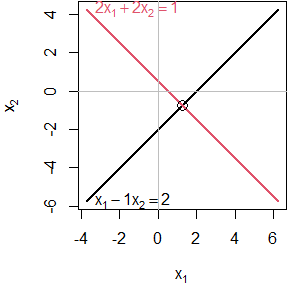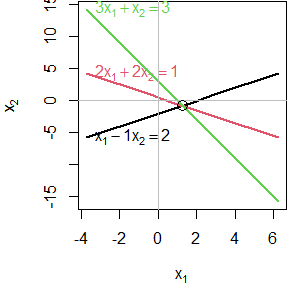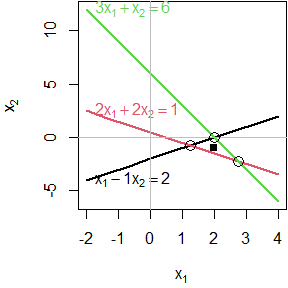# Solving Linear Equations

#### 2021-08-21

This vignette illustrates the ideas behind solving systems of linear equations of the form $$\mathbf{A x = b}$$ where

• $$\mathbf{A}$$ is an $$m \times n$$ matrix of coefficients for $$m$$ equations in $$n$$ unknowns
• $$\mathbf{x}$$ is an $$n \times 1$$ vector unknowns, $$x_1, x_2, \dots x_n$$
• $$\mathbf{b}$$ is an $$m \times 1$$ vector of constants, the “right-hand sides” of the equations

The general conditions for solutions are:

• the equations are consistent (solutions exist) if $$r( \mathbf{A} | \mathbf{b}) = r( \mathbf{A})$$
• the solution is unique if $$r( \mathbf{A} | \mathbf{b}) = r( \mathbf{A}) = n$$
• the solution is underdetermined if $$r( \mathbf{A} | \mathbf{b}) = r( \mathbf{A}) < n$$
• the equations are inconsistent (no solutions) if $$r( \mathbf{A} | \mathbf{b}) > r( \mathbf{A})$$

We use c( R(A), R(cbind(A,b)) ) to show the ranks, and all.equal( R(A), R(cbind(A,b)) ) to test for consistency.

library(matlib)   # use the package

## Equations in two unknowns

Each equation in two unknowns corresponds to a line in 2D space. The equations have a unique solution if all lines intersect in a point.

### Two consistent equations

A <- matrix(c(1, 2, -1, 2), 2, 2)
b <- c(2,1)
showEqn(A, b)
## 1*x1 - 1*x2  =  2
## 2*x1 + 2*x2  =  1
c( R(A), R(cbind(A,b)) )          # show ranks
##  2 2
all.equal( R(A), R(cbind(A,b)) )  # consistent?
##  TRUE

Plot the equations:

plotEqn(A,b)
##   x - 1*x  =  2
## 2*x + 2*x  =  1Solve() is a convenience function that shows the solution in a more comprehensible form:

Solve(A, b, fractions = TRUE)
## x1    =   5/4
##   x2  =  -3/4

### Three consistent equations

For three (or more) equations in two unknowns, $$r(\mathbf{A}) \le 2$$, because $$r(\mathbf{A}) \le \min(m,n)$$. The equations will be consistent if $$r(\mathbf{A}) = r(\mathbf{A | b})$$. This means that whatever linear relations exist among the rows of $$\mathbf{A}$$ are the same as those among the elements of $$\mathbf{b}$$.

Geometrically, this means that all three lines intersect in a point.

A <- matrix(c(1,2,3, -1, 2, 1), 3, 2)
b <- c(2,1,3)
showEqn(A, b)
## 1*x1 - 1*x2  =  2
## 2*x1 + 2*x2  =  1
## 3*x1 + 1*x2  =  3
c( R(A), R(cbind(A,b)) )          # show ranks
##  2 2
all.equal( R(A), R(cbind(A,b)) )  # consistent?
##  TRUE
Solve(A, b, fractions=TRUE)       # show solution 
## x1    =   5/4
##   x2  =  -3/4
##    0  =     0

Plot the equations:

plotEqn(A,b)
##   x - 1*x  =  2
## 2*x + 2*x  =  1
## 3*x   + x  =  3### Three inconsistent equations

Three equations in two unknowns are inconsistent when $$r(\mathbf{A}) < r(\mathbf{A | b})$$.

A <- matrix(c(1,2,3, -1, 2, 1), 3, 2)
b <- c(2,1,6)
showEqn(A, b)
## 1*x1 - 1*x2  =  2
## 2*x1 + 2*x2  =  1
## 3*x1 + 1*x2  =  6
c( R(A), R(cbind(A,b)) )          # show ranks
##  2 3
all.equal( R(A), R(cbind(A,b)) )  # consistent?
##  "Mean relative difference: 0.5"

You can see this in the result of reducing $$\mathbf{A} | \mathbf{b}$$ to echelon form, where the last row indicates the inconsistency.

echelon(A, b)
##      [,1] [,2]  [,3]
## [1,]    1    0  2.75
## [2,]    0    1 -2.25
## [3,]    0    0 -3.00

Solve() shows this more explicitly:

Solve(A, b, fractions=TRUE)
## x1    =  11/4
##   x2  =  -9/4
##    0  =    -3

An approximate solution is sometimes available using a generalized inverse.

x <- MASS::ginv(A) %*% b
x
##      [,1]
## [1,]    2
## [2,]   -1

Plot the equations. You can see that each pair of equations has a solution, but all three do not have a common, consistent solution.

par(mar=c(4,4,0,0)+.1)
plotEqn(A,b, xlim=c(-2, 4))
##   x - 1*x  =  2
## 2*x + 2*x  =  1
## 3*x   + x  =  6
points(x, x, pch=15)## Equations in three unknowns

Each equation in three unknowns corresponds to a plane in 3D space. The equations have a unique solution if all planes intersect in a point.

### Three consistent equations

A <- matrix(c(2, 1, -1,
-3, -1, 2,
-2,  1, 2), 3, 3, byrow=TRUE)
colnames(A) <- paste0('x', 1:3)
b <- c(8, -11, -3)
showEqn(A, b)
##  2*x1 + 1*x2 - 1*x3  =    8
## -3*x1 - 1*x2 + 2*x3  =  -11
## -2*x1 + 1*x2 + 2*x3  =   -3

Are the equations consistent?

c( R(A), R(cbind(A,b)) )          # show ranks
##  3 3
all.equal( R(A), R(cbind(A,b)) )  # consistent?
##  TRUE

Solve for $$\mathbf{x}$$.

solve(A, b)
## x1 x2 x3
##  2  3 -1
solve(A) %*% b
##    [,1]
## x1    2
## x2    3
## x3   -1
inv(A) %*% b
##      [,1]
## [1,]    2
## [2,]    3
## [3,]   -1

Another way to see the solution is to reduce $$\mathbf{A | b}$$ to echelon form. The result is $$\mathbf{I | A^{-1}b}$$, with the solution in the last column.

echelon(A, b)
##      x1 x2 x3
## [1,]  1  0  0  2
## [2,]  0  1  0  3
## [3,]  0  0  1 -1
echelon(A, b, verbose=TRUE, fractions=TRUE)
##
## Initial matrix:
##      x1  x2  x3
## [1,]   2   1  -1   8
## [2,]  -3  -1   2 -11
## [3,]  -2   1   2  -3
##
## row: 1
##
##  exchange rows 1 and 2
##      x1  x2  x3
## [1,]  -3  -1   2 -11
## [2,]   2   1  -1   8
## [3,]  -2   1   2  -3
##
##  multiply row 1 by -1/3
##      x1   x2   x3
## [1,]    1  1/3 -2/3 11/3
## [2,]    2    1   -1    8
## [3,]   -2    1    2   -3
##
##  multiply row 1 by 2 and subtract from row 2
##      x1   x2   x3
## [1,]    1  1/3 -2/3 11/3
## [2,]    0  1/3  1/3  2/3
## [3,]   -2    1    2   -3
##
##  multiply row 1 by 2 and add to row 3
##      x1   x2   x3
## [1,]    1  1/3 -2/3 11/3
## [2,]    0  1/3  1/3  2/3
## [3,]    0  5/3  2/3 13/3
##
## row: 2
##
##  exchange rows 2 and 3
##      x1   x2   x3
## [1,]    1  1/3 -2/3 11/3
## [2,]    0  5/3  2/3 13/3
## [3,]    0  1/3  1/3  2/3
##
##  multiply row 2 by 3/5
##      x1   x2   x3
## [1,]    1  1/3 -2/3 11/3
## [2,]    0    1  2/5 13/5
## [3,]    0  1/3  1/3  2/3
##
##  multiply row 2 by 1/3 and subtract from row 1
##      x1   x2   x3
## [1,]    1    0 -4/5 14/5
## [2,]    0    1  2/5 13/5
## [3,]    0  1/3  1/3  2/3
##
##  multiply row 2 by 1/3 and subtract from row 3
##      x1   x2   x3
## [1,]    1    0 -4/5 14/5
## [2,]    0    1  2/5 13/5
## [3,]    0    0  1/5 -1/5
##
## row: 3
##
##  multiply row 3 by 5
##      x1   x2   x3
## [1,]    1    0 -4/5 14/5
## [2,]    0    1  2/5 13/5
## [3,]    0    0    1   -1
##
##  multiply row 3 by 4/5 and add to row 1
##      x1   x2   x3
## [1,]    1    0    0    2
## [2,]    0    1  2/5 13/5
## [3,]    0    0    1   -1
##
##  multiply row 3 by 2/5 and subtract from row 2
##      x1 x2 x3
## [1,]  1  0  0  2
## [2,]  0  1  0  3
## [3,]  0  0  1 -1

Plot them. plotEqn3d uses rgl for 3D graphics. If you rotate the figure, you’ll see an orientation where all three planes intersect at the solution point, $$\mathbf{x} = (2, 3, -1)$$

plotEqn3d(A,b, xlim=c(0,4), ylim=c(0,4))

### Three inconsistent equations

A <- matrix(c(1,  3, 1,
1, -2, -2,
2,  1, -1), 3, 3, byrow=TRUE)
colnames(A) <- paste0('x', 1:3)
b <- c(2, 3, 6)
showEqn(A, b)
## 1*x1 + 3*x2 + 1*x3  =  2
## 1*x1 - 2*x2 - 2*x3  =  3
## 2*x1 + 1*x2 - 1*x3  =  6

Are the equations consistent? No.

c( R(A), R(cbind(A,b)) )          # show ranks
##  2 3
all.equal( R(A), R(cbind(A,b)) )  # consistent?
##  "Mean relative difference: 0.5"